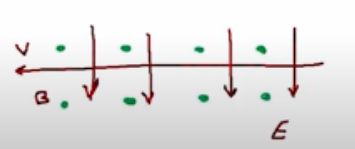Need Help?

Subscribe to Physics 2

###### \${selected_topic_name}
• Notes
• Comments & Questions

$\begin{array}{l}{\text { (a) What is the speed of a beam of electrons when the }} \\ {\text { simultaneous influence of an electric field of } 1.56 \times 10^{4} \mathrm{V} / \mathrm{m} \text { and }} \\ {\text { a magnetic field of } 4.62 \times 10^{-3} \mathrm{T} \text { , with both fields normal to the }} \\ {\text { beam and to each other, produces no deflection of the electrons? }} \\ {\text { (b) In a diagram, show the relative orientation of the vectors }} \\ {\vec{v}, \vec{E}, \text { and } \vec{B} \text { . (c) When the electric field is removed, what is the }} \\ {\text { radius of the electron orbit? What is the period of the orbit? }}\end{array}$

$V=\frac{E}{B}$

$|q| V B=\frac{m v^{2}}{R}$

(a) $V=\frac{E}{B}=\frac{1.56*10^{4}}{4.62 * 10^{-3}}=3.38 * 10^{6} \mathrm{m} / \mathrm{s}$

(b)(c) $R=\frac{m v}{|q| B}$$=\frac{9.11 * 10^{-31} * 3.38 * 10^{6}}{1.6 * 10^{-19}*4.62 * 10^{-3}}$

$=4.17*10^{-3} \mathrm{m}$

$T=\frac{2 \pi m}{|q|B}$$=\frac{2 \pi R}{V}= \frac{2 \pi\left(4.17*10^{-3}\right)}{3.38 * 10^{6}}=7.74 * 10^{-9} \mathrm{s}$

$\begin{array}{l}{\text { Singly ionized (one electron removed) atoms are acceler- }} \\ {\text { ated and then passed through a velocity selector consisting of per- }} \\ {\text { pendicular electric and magnetic fields. The electric field is } 155 \mathrm{V} / \mathrm{m}} \\ {\text { and the magnetic field is } 0.0315 \mathrm{T} \text { . The ions next enter a uniform }} \\ {\text { magnetic field of magnitude } 0.0175 \text { T that is oriented perpendicu- }}\end{array}$

$\begin{array}{l}{\text { lar to their velocity. (a) How fast are the ions moving when they }} \\ {\text { emerge from the velocity selector? (b) If the radius of the path of }} \\ {\text { the ions in the second magnetic field is } 17.5 \mathrm{cm}, \text { what is their }} \\ {\text { mass? }}\end{array}$

$V=\frac{E}{B}$

$\rightarrow R=\frac{m v}{|q| B}$

$+e$

(a) $V=\frac{E}{B}=\frac{155}{0.0315}=4.92 * 10^{3} \mathrm{m/s}$

(b) $m=\frac{R|q| B}{V}=$$\frac{0.175*1.6 * 10^{-19} * 0.0175}{4.92 * 10^{3}}$$=9.96 * 10^{-26} \mathrm{kg}$

No comments yet